Скачать презентацию Multivariate Digital Signature Schemes Jiun-Ming Chen http www

• Количество слайдов: 38Multivariate Digital Signature Schemes Jiun-Ming Chen http: //www. math. ntu. edu. tw/~jmchen 1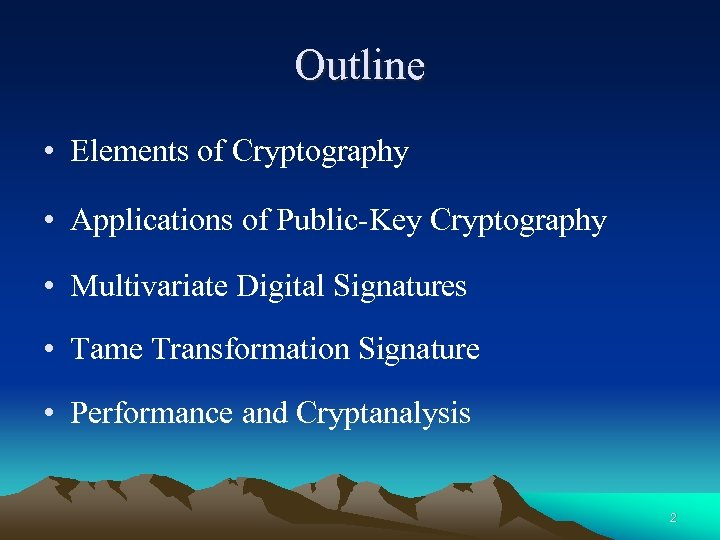Outline • Elements of Cryptography • Applications of Public-Key Cryptography • Multivariate Digital Signatures • Tame Transformation Signature • Performance and Cryptanalysis 2Basics • A cryptosystem consists of an algorithm, all possible keys, plaintexts, and ciphertexts. • Its security is based on the privacy of its keys, not the privacy of its algorithm. • In math language: the type of the function known, but its parameters are secret. is 3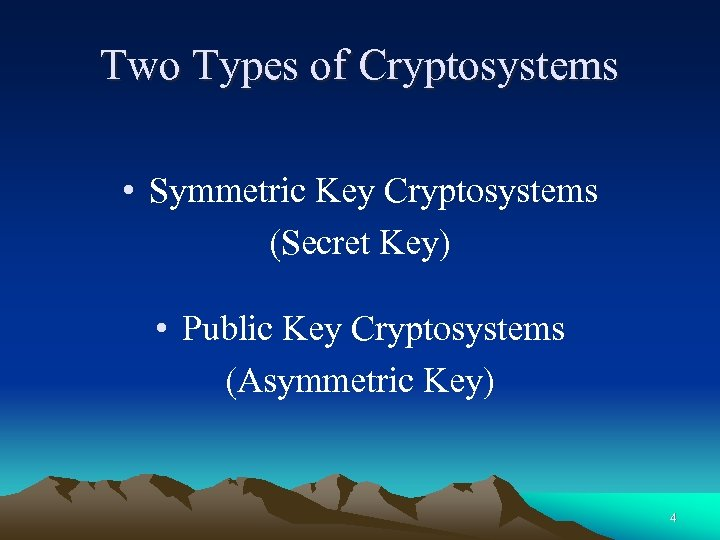Two Types of Cryptosystems • Symmetric Key Cryptosystems (Secret Key) • Public Key Cryptosystems (Asymmetric Key) 4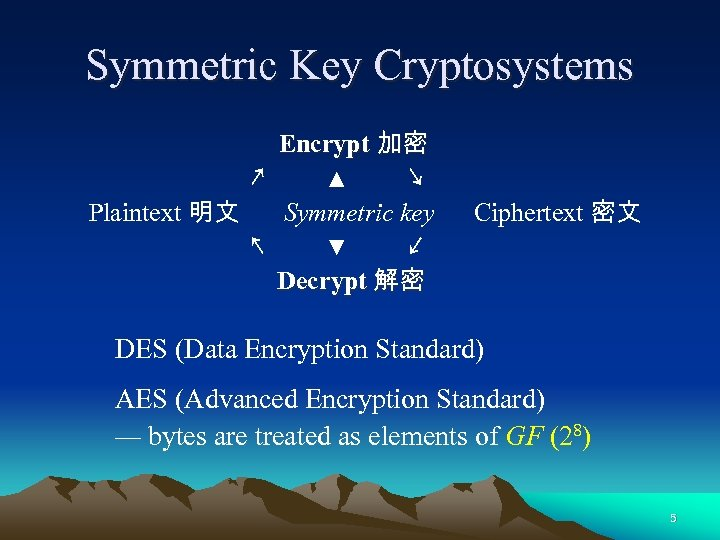Symmetric Key Cryptosystems Encrypt 加密 ↗ ▲ ↘ Plaintext 明文 Symmetric key ↖ ▼ ↙ Decrypt 解密 Ciphertext 密文 DES (Data Encryption Standard) AES (Advanced Encryption Standard) — bytes are treated as elements of GF (28) 5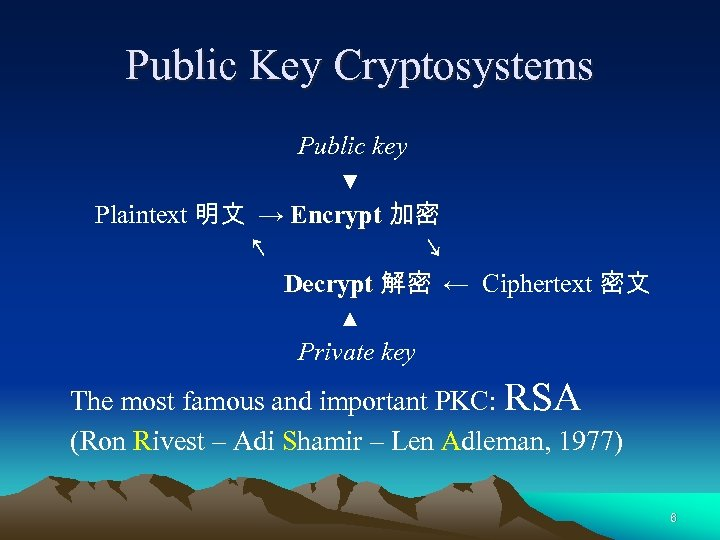Public Key Cryptosystems Public key ▼ Plaintext 明文 → Encrypt 加密 ↖ ↘ Decrypt 解密 ← Ciphertext 密文 ▲ Private key The most famous and important PKC: RSA (Ron Rivest – Adi Shamir – Len Adleman, 1977) 6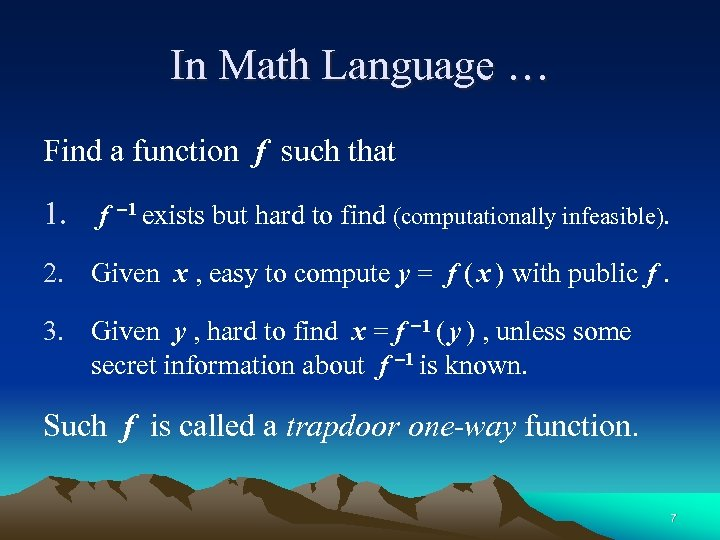In Math Language … Find a function f such that 1. f 1 exists but hard to find (computationally infeasible). 2. Given x , easy to compute y = f ( x ) with public f. 3. Given y , hard to find x = f 1 ( y ) , unless some secret information about f 1 is known. Such f is called a trapdoor one-way function. 7Digital Signatures 數位簽章 Private key 私鑰 ▼ Message → Sign 簽章 ↖ ↘ Verify 驗章 ← Signature ▲ Public key 公鑰 8Public Key Infrastructure • CA (Certificate Authority) – 憑證管理中心 RA (Registration Authority) – 憑證註冊中心 • Confidentiality (秘密性 ) Authentication (身份鑑別性 ) Integrity (完整性 ) Non-repudiation (不可否認性 ) • 數位簽章 是 公開金鑰基礎建設 ( PKI )的核心技術 9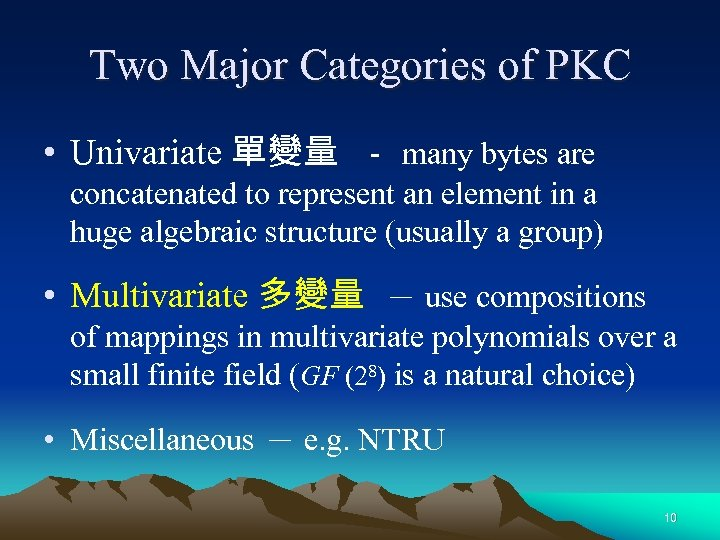Two Major Categories of PKC • Univariate 單變量 － many bytes are concatenated to represent an element in a huge algebraic structure (usually a group) • Multivariate 多變量 － use compositions of mappings in multivariate polynomials over a small finite field (GF (28) is a natural choice) • Miscellaneous － e. g. NTRU 10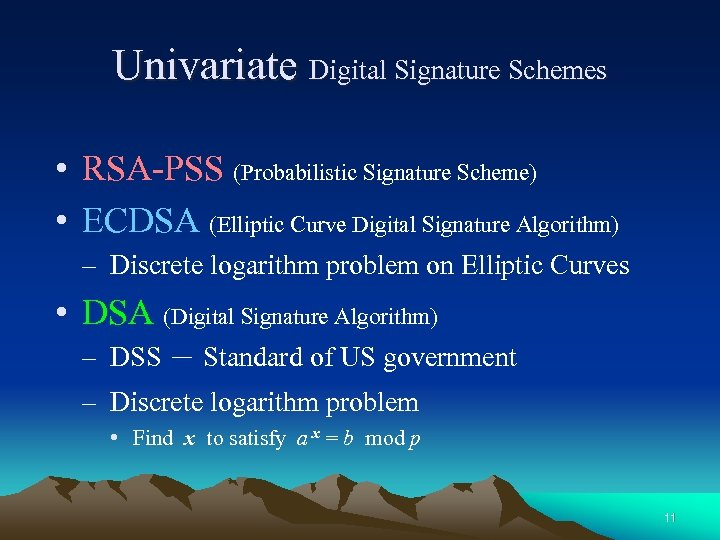Univariate Digital Signature Schemes • RSA-PSS (Probabilistic Signature Scheme) • ECDSA (Elliptic Curve Digital Signature Algorithm) – Discrete logarithm problem on Elliptic Curves • DSA (Digital Signature Algorithm) – DSS － Standard of US government – Discrete logarithm problem • Find x to satisfy a x = b mod p 11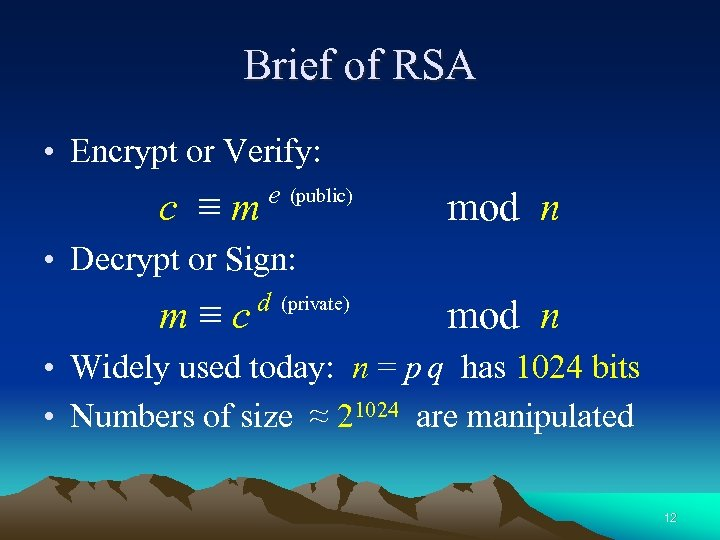Brief of RSA • Encrypt or Verify: c ≡m e (public) mod n • Decrypt or Sign: m≡c d (private) mod n • Widely used today: n = p q has 1024 bits • Numbers of size ≈ 21024 are manipulated 12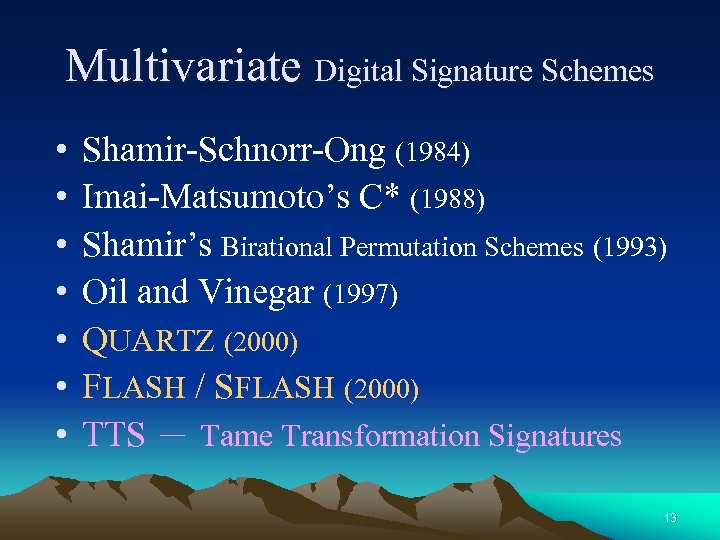Multivariate Digital Signature Schemes • • Shamir-Schnorr-Ong (1984) Imai-Matsumoto’s C* (1988) Shamir’s Birational Permutation Schemes (1993) Oil and Vinegar (1997) QUARTZ (2000) FLASH / SFLASH (2000) TTS － Tame Transformation Signatures 13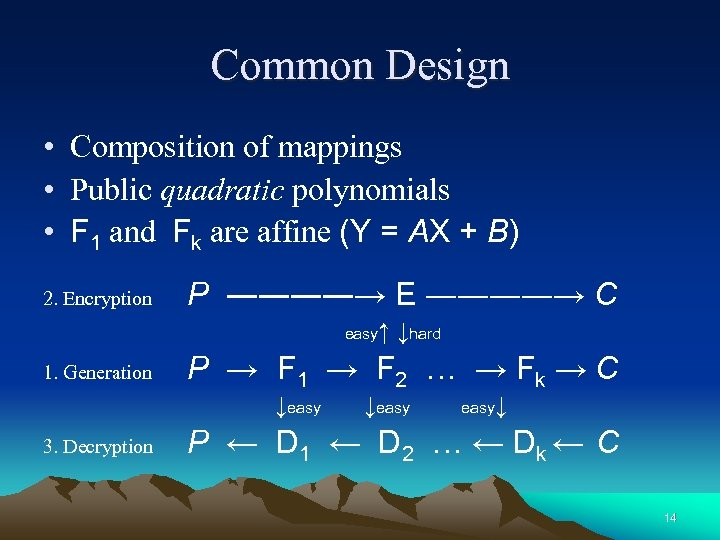Common Design • Composition of mappings • Public quadratic polynomials • F 1 and Fk are affine (Y = AX + B) 2. Encryption P ――――→ E ――――→ C easy↑ 1. Generation P → F 1 → F 2 … → Fk → C ↓easy 3. Decryption ↓hard ↓easy↓ P ← D 1 ← D 2 … ← Dk ← C 14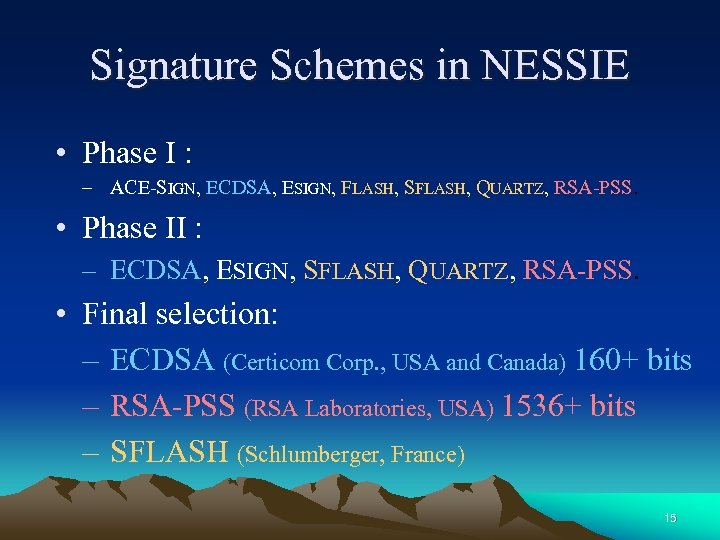Signature Schemes in NESSIE • Phase I : – ACE-SIGN, ECDSA, ESIGN, FLASH, SFLASH, QUARTZ, RSA-PSS. • Phase II : – ECDSA, ESIGN, SFLASH, QUARTZ, RSA-PSS. • Final selection: – ECDSA (Certicom Corp. , USA and Canada) 160+ bits – RSA-PSS (RSA Laboratories, USA) 1536+ bits – SFLASH (Schlumberger, France) 15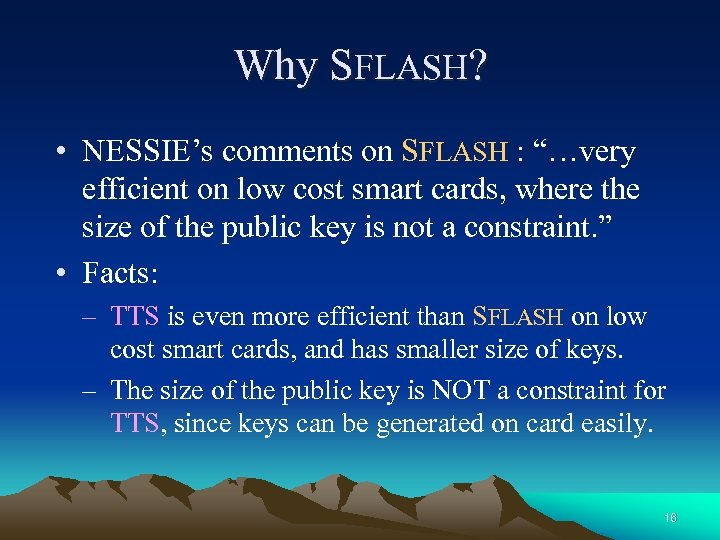Why SFLASH? • NESSIE’s comments on SFLASH : “…very efficient on low cost smart cards, where the size of the public key is not a constraint. ” • Facts: – TTS is even more efficient than SFLASH on low cost smart cards, and has smaller size of keys. – The size of the public key is NOT a constraint for TTS, since keys can be generated on card easily. 16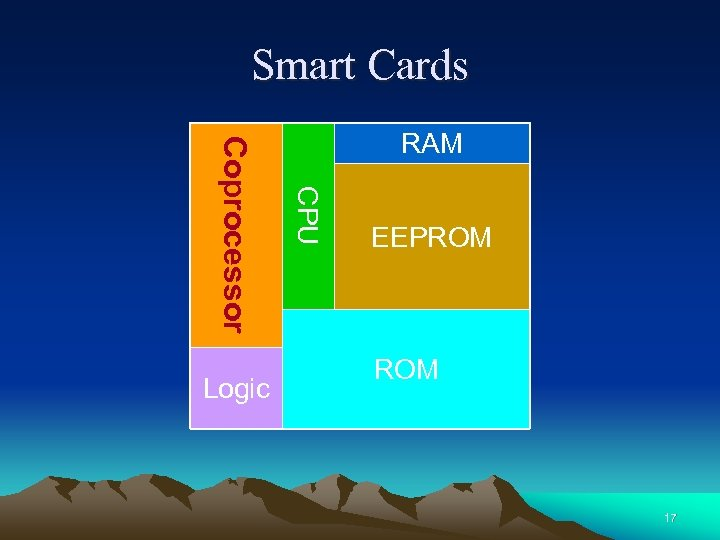Smart Cards CPU Coprocessor Logic RAM EEPROM 17Comparison on Pentium III/500 Scheme ECDSA (163 bits) RSA-PSS (1024 bits) SFLASH (26, 37) TTS (20, 28) Key Setup 1. 6 ms 2. 7 sec 1. 5 sec 15. 8 ms Signing 1. 9 ms 84 ms 2. 8 ms 0. 045 ms Verifying 5. 1 ms 2. 0 ms 0. 39 ms 0. 25 ms Signature Size 326 bits 1024 bits 259 bits 224 bits Public Key Size 48 bytes 128 bytes 15. 4 KB 8. 6 KB Private Key Size 24 bytes 320 bytes 2. 4 KB 1. 4 KB Data of ECDSA, RSA-PSS, and SFLASH from NESSIE Performance Report 18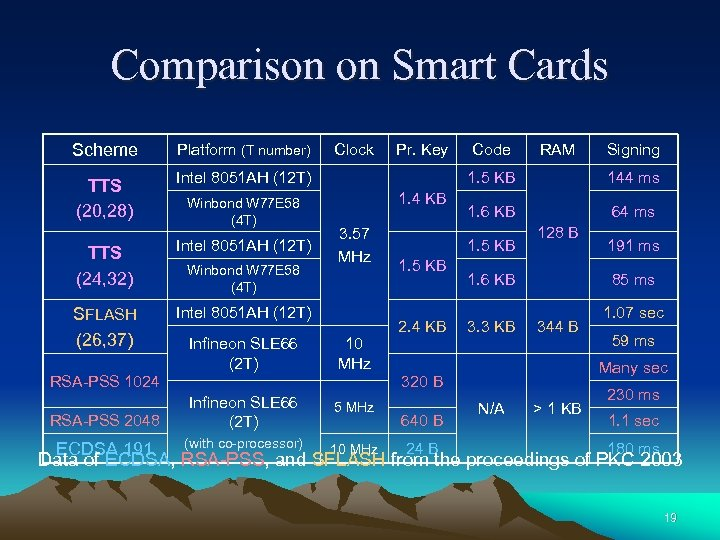Comparison on Smart Cards Scheme Platform (T number) TTS (20, 28) Intel 8051 AH (12 T) TTS (24, 32) Intel 8051 AH (12 T) SFLASH (26, 37) Intel 8051 AH (12 T) RSA-PSS 1024 RSA-PSS 2048 ECDSA 191 Winbond W 77 E 58 (4 T) Infineon SLE 66 (2 T) Clock Pr. Key Code RAM Signing 1. 5 KB 1. 4 KB 3. 57 MHz 10 MHz Infineon SLE 66 (2 T) 5 MHz (with co-processor) 10 MHz 144 ms 1. 6 KB 64 ms 1. 5 KB 2. 4 KB 128 B 1. 6 KB 3. 3 KB 85 ms 344 B 24 B 1. 07 sec 59 ms Many sec 320 B 640 B 191 ms N/A > 1 KB 230 ms 1. 1 sec 180 ms Data of ECDSA, RSA-PSS, and SFLASH from the proceedings of PKC 2003 19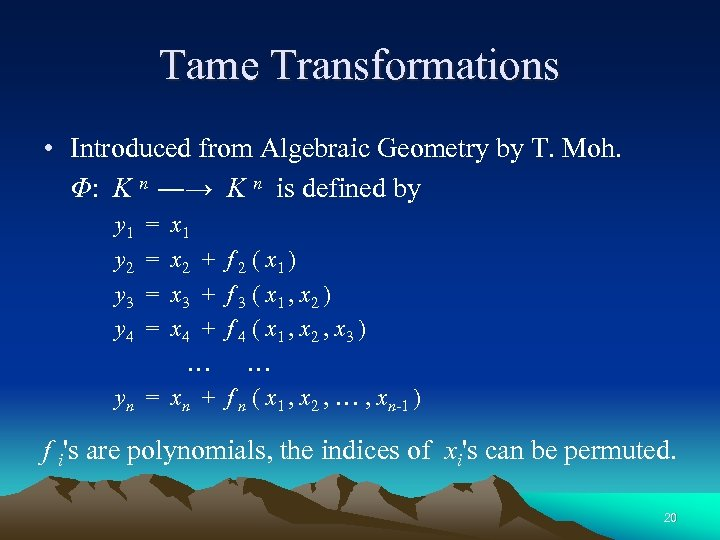Tame Transformations • Introduced from Algebraic Geometry by T. Moh. Φ: K n ―→ K n is defined by y 1 y 2 y 3 y 4 = = x 1 x 2 + x 3 + x 4 + … yn = xn + f 2 ( x 1 ) f 3 ( x 1 , x 2 ) f 4 ( x 1 , x 2 , x 3 ) … f n ( x 1 , x 2 , … , xn-1 ) f i's are polynomials, the indices of xi's can be permuted. 20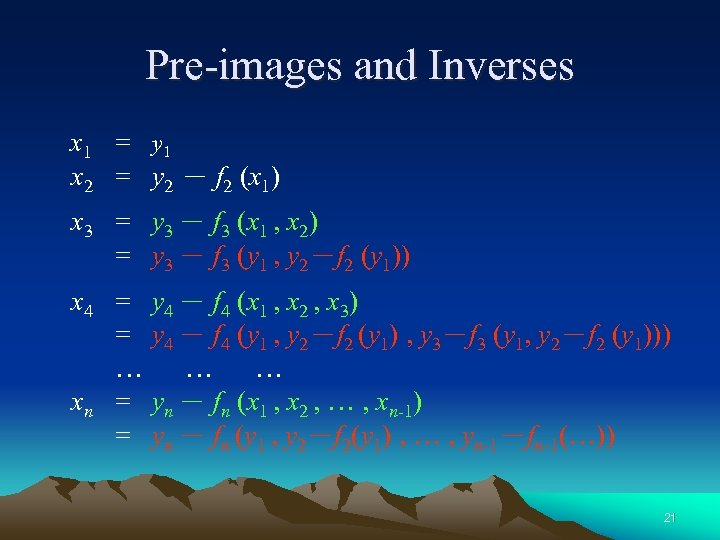Pre-images and Inverses x 1 = y 1 x 2 = y 2 － f 2 (x 1) x 3 = y 3 － f 3 (x 1 , x 2) = y 3 － f 3 (y 1 , y 2－f 2 (y 1)) x 4 = = … xn = = y 4 － f 4 (x 1 , x 2 , x 3) y 4 － f 4 (y 1 , y 2－f 2 (y 1) , y 3－f 3 (y 1, y 2－f 2 (y 1))) … … yn － fn (x 1 , x 2 , … , xn-1) yn － fn (y 1 , y 2－f 2(y 1) , … , yn-1－fn-1(…)) 21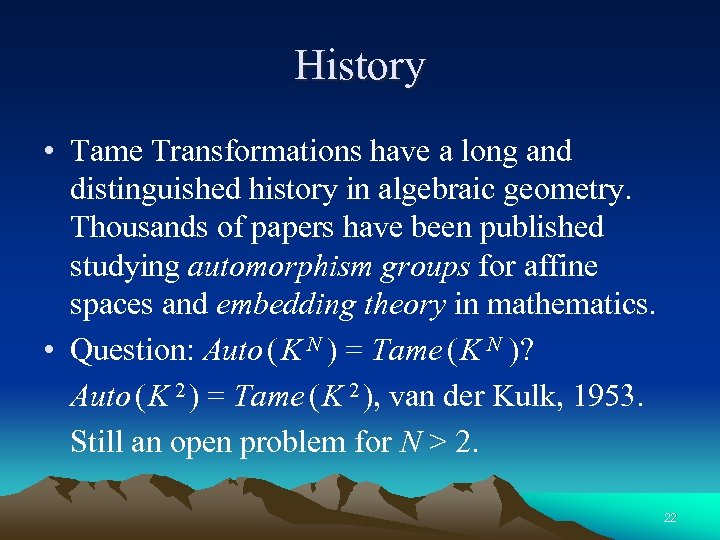History • Tame Transformations have a long and distinguished history in algebraic geometry. Thousands of papers have been published studying automorphism groups for affine spaces and embedding theory in mathematics. • Question: Auto ( K N ) = Tame ( K N )? Auto ( K 2 ) = Tame ( K 2 ), van der Kulk, 1953. Still an open problem for N > 2. 22Factorization in Tame ( K N ) • Given an element π Tame ( K N ) , N > 2. No known way to factor π= φt。 。φ1. That is, no factorization theorem for N > 2. • Nagata’s example, 1972: y 1 = x 1 y 2 = x 2 + x 1 ( x 1 x 3 + x 22 ) y 3 = x 3 − x 2 ( x 1 x 3 + x 22 ) − x 1 ( x 1 x 3 + x 22 ) 2 Is it in Tame ( K 3 )? Nobody can answer yet. 23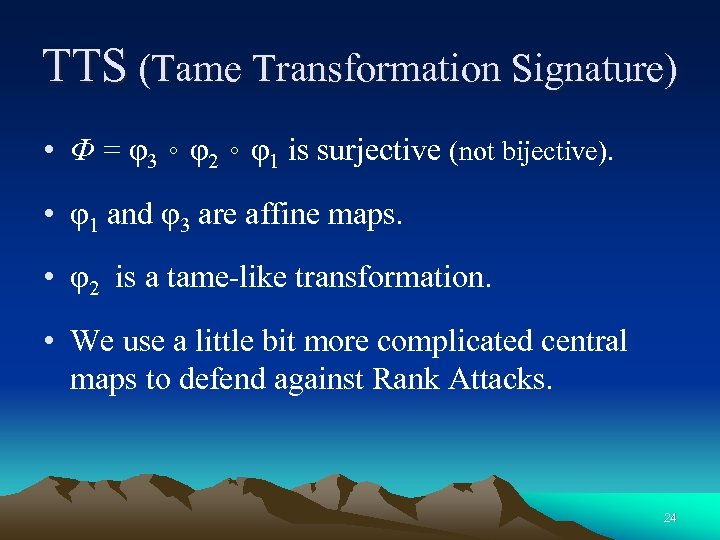TTS (Tame Transformation Signature) • Φ = φ3。φ2。φ1 is surjective (not bijective). • φ1 and φ3 are affine maps. • φ2 is a tame-like transformation. • We use a little bit more complicated central maps to defend against Rank Attacks. 24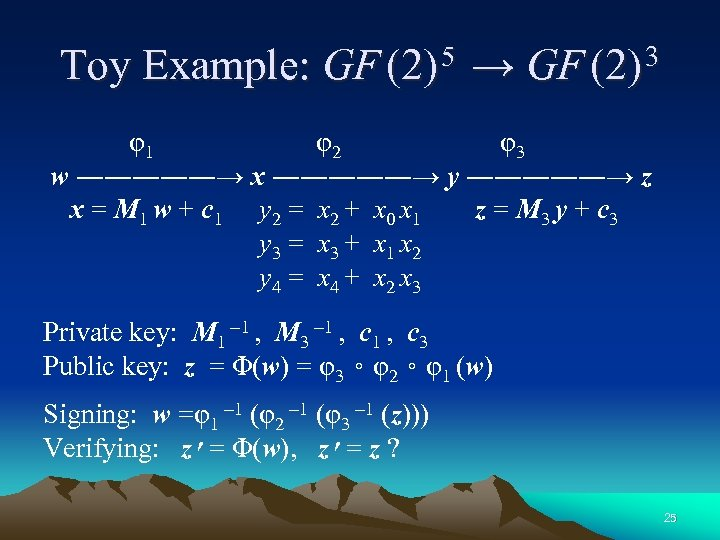Toy Example: GF (2) 5 → GF (2) 3 φ1 φ2 φ3 w ―――――→ x ―――――→ y ―――――→ z x = M 1 w + c 1 y 2 = x 2 + x 0 x 1 z = M 3 y + c 3 y 3 = x 3 + x 1 x 2 y 4 = x 4 + x 2 x 3 Private key: M 1 1 , M 3 1 , c 3 Public key: z = Φ(w) = φ3。φ2。φ1 (w) Signing: w =φ1 1 (φ2 1 (φ3 1 (z))) Verifying: z = ׳ Φ(w), z = ׳ z ? 25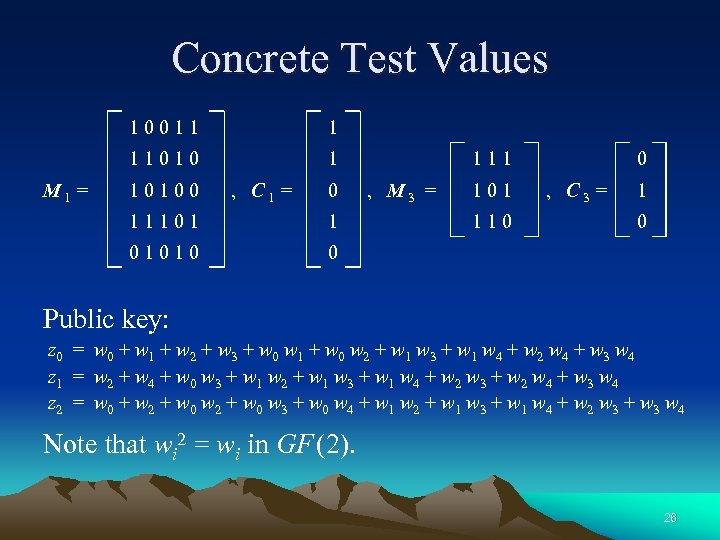Concrete Test Values 10011 11010 M 1 = 1 1 10100 , C 1 = 0 11101 1 01010 111 , M 3 = 101 110 0 , C 3 = 1 0 0 Public key: z 0 = w 0 + w 1 + w 2 + w 3 + w 0 w 1 + w 0 w 2 + w 1 w 3 + w 1 w 4 + w 2 w 4 + w 3 w 4 z 1 = w 2 + w 4 + w 0 w 3 + w 1 w 2 + w 1 w 3 + w 1 w 4 + w 2 w 3 + w 2 w 4 + w 3 w 4 z 2 = w 0 + w 2 + w 0 w 3 + w 0 w 4 + w 1 w 2 + w 1 w 3 + w 1 w 4 + w 2 w 3 + w 3 w 4 Note that wi 2 = wi in GF (2). 26Signing a Mini Message (1/3) φ1 1 φ2 1 φ3 1 w ←――――― x ←――――― y ←――――― z x = M 1 w + c 1 1 = y 2 = x 2 + x 0 x 1 z = M 3 y + c 3 1 = y 3 = x 3 + x 1 x 2 y = M 3 1 (z c 3) 1 = y 4 = x 4 + x 2 x 3 • Assume a mini message to sign: z = (1, 1, 0). • Then y = M 3 1 (z c 3) = (1, 1, 1). 27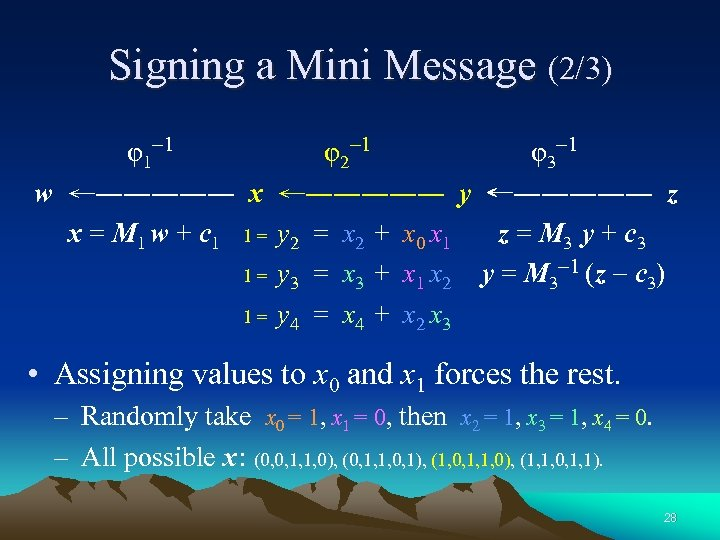Signing a Mini Message (2/3) φ1 1 φ2 1 φ3 1 w ←――――― x ←――――― y ←――――― z x = M 1 w + c 1 1 = y 2 = x 2 + x 0 x 1 z = M 3 y + c 3 1 = y 3 = x 3 + x 1 x 2 y = M 3 1 (z c 3) 1 = y 4 = x 4 + x 2 x 3 • Assigning values to x 0 and x 1 forces the rest. – Randomly take x 0 = 1, x 1 = 0, then x 2 = 1, x 3 = 1, x 4 = 0. – All possible x : (0, 0, 1, 1, 0), (0, 1, 1, 0, 1), (1, 0, 1, 1, 0), (1, 1, 0, 1, 1). 28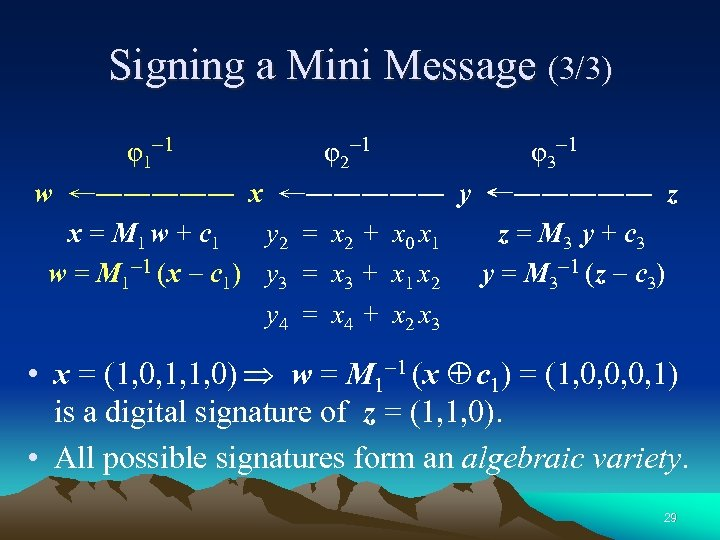Signing a Mini Message (3/3) φ1 1 φ2 1 φ3 1 w ←――――― x ←――――― y ←――――― z x = M 1 w + c 1 y 2 = x 2 + x 0 x 1 z = M 3 y + c 3 w = M 1 1 (x c 1) y 3 = x 3 + x 1 x 2 y = M 3 1 (z c 3) y 4 = x 4 + x 2 x 3 • x = (1, 0, 1, 1, 0) w = M 1 1 (x c 1) = (1, 0, 0, 0, 1) is a digital signature of z = (1, 1, 0). • All possible signatures form an algebraic variety. 29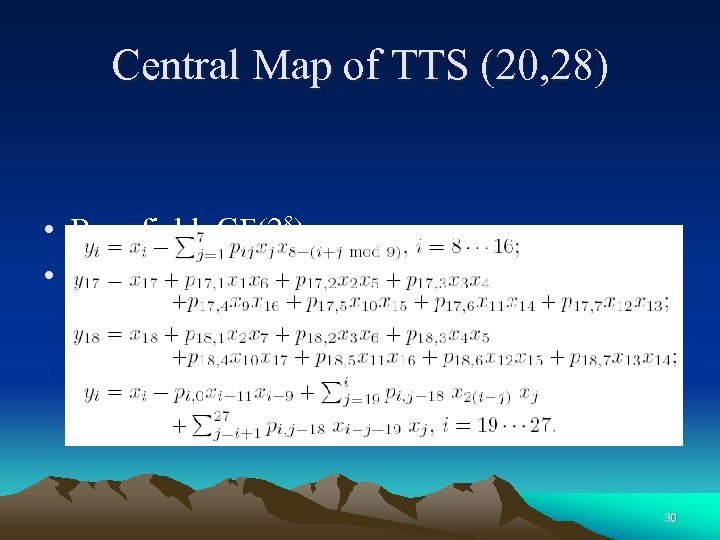Central Map of TTS (20, 28) • Base field: GF(28) • Central map: 30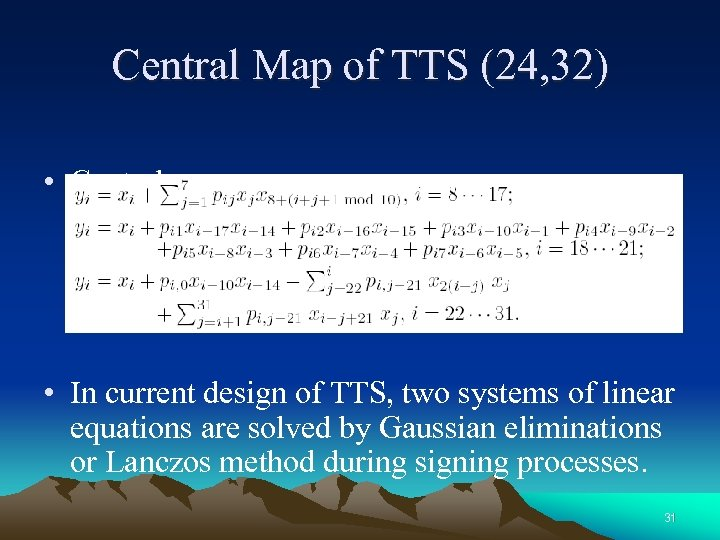Central Map of TTS (24, 32) • Central map: • In current design of TTS, two systems of linear equations are solved by Gaussian eliminations or Lanczos method during signing processes. 31Related Attacks • Various Rank Attacks – Low rank attack – High rank attack (Dual rank attack) – Separation of variables (Unbalanced Oil and Vinegar) • System of Equations Solving Methods – Gröbner bases – Family of XL, XL , FXL, . . . 32Forging a Digital Signature • Given z = (z 1, …, zm), forging a signature is equivalent to finding a solution w = (w 1, …, wn) to the system of equations z = Φ(w). That is, zk = Σi < j pi j k wi wj +Σqj k wj 2 +Σrj k wj for every k. • Fact: Solving a large system of multivariate quadratic equations over GF (q) is NP-hard. 33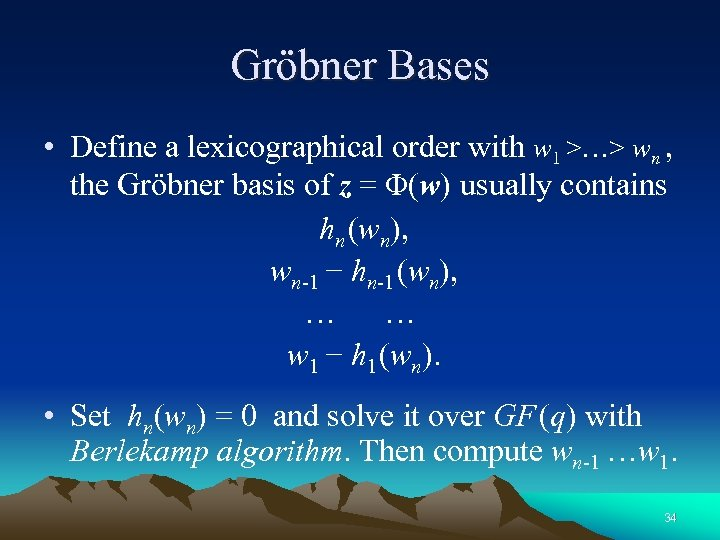Gröbner Bases • Define a lexicographical order with w 1 >…> wn , the Gröbner basis of z = Φ(w) usually contains hn (wn), wn-1 − hn-1 (wn), … … w 1 − h 1 (wn). • Set hn(wn) = 0 and solve it over GF (q) with Berlekamp algorithm. Then compute wn-1 …w 1. 34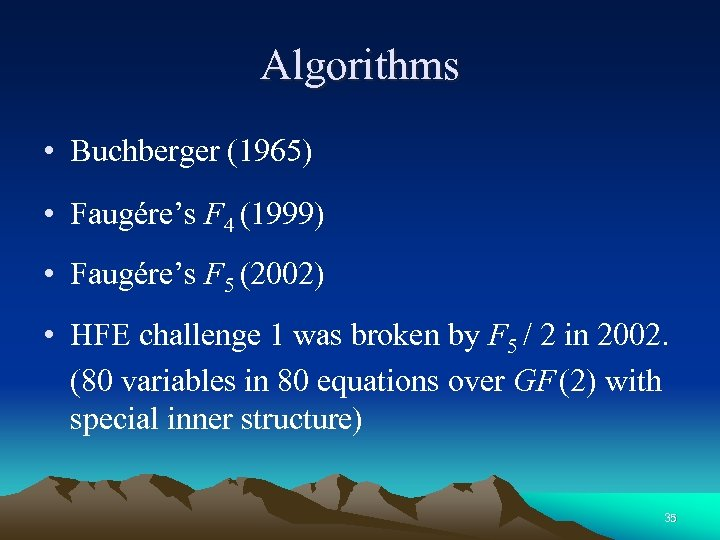Algorithms • Buchberger (1965) • Faugére’s F 4 (1999) • Faugére’s F 5 (2002) • HFE challenge 1 was broken by F 5 / 2 in 2002. (80 variables in 80 equations over GF (2) with special inner structure) 35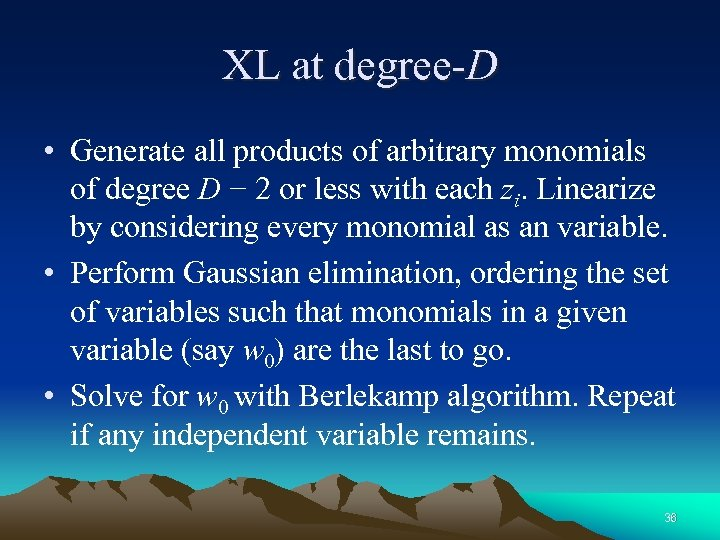XL at degree-D • Generate all products of arbitrary monomials of degree D − 2 or less with each zi. Linearize by considering every monomial as an variable. • Perform Gaussian elimination, ordering the set of variables such that monomials in a given variable (say w 0) are the last to go. • Solve for w 0 with Berlekamp algorithm. Repeat if any independent variable remains. 36Mathematics Connected to XL • Combinatorics – Gives formulas for parameter D 0 (minimal D needed by XL) for generic cases. • Algebra – Gives results on behavior of non-generic system, including Lemma of Operability. Of particular interest is Fröberg’s “Maximal Rank Conjecture”. • Analysis – Gives asymptotic estimates for XL and variants. 37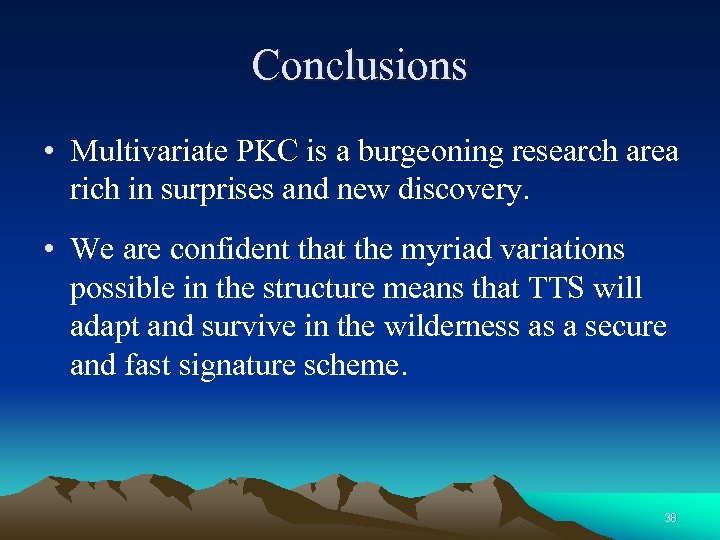Conclusions • Multivariate PKC is a burgeoning research area rich in surprises and new discovery. • We are confident that the myriad variations possible in the structure means that TTS will adapt and survive in the wilderness as a secure and fast signature scheme. 38